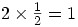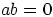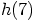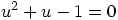Warning: Illegal string offset 'html' in /home/hsn/public_html/forum/cache/skin_cache/cacheid_1/skin_topic.php on line 909

Compund Angle Formulae Homework - HSN forumCompund Angle Formulae Homework

12 replies to this topic

#1d2vid

• Location:Very Dark Side of the Internet
• Interests:Computers, Graphics, vBulletin, PHP, Hacking, Web scripts, Maths, and more...
• Gender:Male

Posted 11 January 2007 - 11:19 AM

hello everyoneI am not really sure about questions 5 b,c, because there is no numbers, so i cannot really solve it. All I can do is to change the style of the question.

e.g. 5b) 3sin2x + 2cosx = 0 ->> 3(2sinxcosx) + 2cosx = 0 ->> 6sinx3cosx + 2cosx = 0 ... and that's how far i've got..

in 5c) i change cos2x to 1-sinx and that's all?

another one is question 7.. I think that t should equal 30 to get the lowest point (like on the clock, because there is 60 seconds) and my theory is imo kind of correct.. when i use t = 30 i get 1.13m, when i use 60 i get 2.86m and when i use 0 i get 2.86m so i think it is a highest point..
however.. when i use t = 45 i get 2.5m and if i use t = 15 i get 1.5m and this isn't correct, because these points should be at the same height. Also i used t = 31 and i get the lowest point 1m.. and i am completely confused now.. because if it is seconds.. then it should be divided for 60 parts (60 seconds).. but i don't know.. so i'm asking for help.

CODE
http://rapidshare.com/files/11196049/compound_angle_formulae.pdf.html
http://www.sendspace.com/file/35w29o

Thanks in advance"Imagination is more important than knowledge"
---------------------------------------------
Going to Uni next year!

#2Untouchable

• Gender:Male

Posted 11 January 2007 - 01:17 PM

5b. 3sin2x + 2cosx = 0

->> 3(2sinxcosx) + 2cosx = 0
->> 6sinxcosx + 2cosx = 0 Yours was incorrect here!
TAKE OUT 2cosx AS COMMON FACTOR
->> 2cosx(3sinx + 1)= 0

Therefore 2cosx = 0 or 3sinx + 1 = 0 Solve these basic trig equations

#3d2vid

• Location:Very Dark Side of the Internet
• Interests:Computers, Graphics, vBulletin, PHP, Hacking, Web scripts, Maths, and more...
• Gender:Male

Posted 11 January 2007 - 04:08 PM

aye.. thanks @Untouchable.. now waiting for the other answers.. cuz the homework is due tomorrow.
"Imagination is more important than knowledge"
---------------------------------------------
Going to Uni next year!

#4Moondarra

• Location:Fraserburgh
• Interests:Maths, playing guitar and of course music. chess and anything a challenge
• Gender:Male

Posted 11 January 2007 - 05:36 PM

Q5c cos2x - sinx = 0 0x2cos2x = 1 - sin2x

hence

1 - sin2x - sinx = 0
-(sin2x + sinx - 1) = 0

you should be able to do it from there, just factore and you have you answers

Q7a the minimum height of the graph is two above the axis so therefore QR = 2

b)

the particle will reach point q when its at it minimum height theis happens when sin(30t + 60) = 0

30t + 60 = sin-10
30t + 60 = 0 OR 30t + 60 = 360
30t = -60 OR 30t = 300
t = -2 OR t = 10

we reject t = -2 as a negative time is impossible and therefore it r4eaches point Q at t = 10

#5d2vid

• Location:Very Dark Side of the Internet
• Interests:Computers, Graphics, vBulletin, PHP, Hacking, Web scripts, Maths, and more...
• Gender:Male

Posted 11 January 2007 - 05:51 PM

thanks @Moondarra,

in 5c) (just to confirm)

sin^2x + sinx = 1 ->> sinx (sinx + 1) = 1 ->> sinx = 1 or sinx = 0

and about question 7... sinx = 0 ->> x = 0, 180 or 360 i think, because it touches x-axis on 0, 180 and 360.. so should i also do the equation for 180?
"Imagination is more important than knowledge"
---------------------------------------------
Going to Uni next year!

#6Steve

Top of the Class

••• 435 posts
• Location:Edinburgh
• Gender:Male

Posted 11 January 2007 - 06:29 PM

in 5c) (just to confirm)

sin^2x + sinx = 1 ->> sinx (sinx + 1) = 1 ->> sinx = 1 or sinx = 0

That's not right, if you have two things multiplied together equal to 1, this doesn't mean that one of them has to be 1, e.g.but neither 2 nor 1/2 are equal to 1!.

You can only do this sort of thing when you have something factorised equal to zero, because ifthen either a or b must be zero (you can't multiply to non-zero things and get zero).

I don't agree that QR=2 in Q7, since the minimum value of the sine function is -1, so the minimum distance between the axis and a point on the circle is 1, which is what QR is. (You can easily check that 2 is wrong by evaluating.)
HSN contribute: Help the site grow!

Looking for a Maths tutor in West Lothian? Just PM me!

Top of the Class

•• Members
•• 390 posts
• Location:Cambridge
• Interests:Muzak.
• Gender:Male

Posted 11 January 2007 - 06:33 PM

thanks @Moondarra,

in 5c) (just to confirm)

sin^2x + sinx = 1 ->> sinx (sinx + 1) = 1 ->> sinx = 1 or sinx = 0
Not quite I'm afraid. You have to solve the quadratic equation sin^2x + sinx - 1 =0 (use the quadratic formula). What you have said (if sinx(sinx+1)=1 then either sinx = 1 or sinx+1=1) is tricky business -you can't say anything about a*b=1 unless you're absolutely sure a and b are integers (then either a=b=1 or a=b=-1). Here though, sinx and sinx + 1 are almost never integers (only at multiples of pi/2 actually), so it's best to solve it as a normal quadratic.

Note that equations to zero are always good, because then you know that at least one factor is zero. Equations to some non-zero integer are unlikely to come up at higher/advanced higher though (maybe in the maths challenge or BMO though).

By the way, you should always sub in your values into the original equation to check. Neither x=0 nor x=/2 satisfy the original equation.

For 7. I'm afraid you are looking at this wrong. You have a function for the height after a time T. From looking at the picture you should see that QR is the minimum value of this function. You could differentiate it or whatever, but this is a waste of time. Look at the function:
h(t) = 2 + sin(30t + 60)
the minimum value of the function is when the sin part is at it's least, i.e. sin(30t + 60)=-1. This means that:
h(t) = 2-1 = 1

For the next part, all you are doing is finding the value of t when the particle reaches Q. From the first part of the question, you know that the height of the particle above the ground is QR=1. So all you are doing is saying:
h(t) = 1 = 2+sin(30t + 60)
==> sin(30t + 60) = -1
Can you solve this?

Edit - Too slowListen to Steve anyway, he makes things clearer than meHMFC - Founded 1874, beefing the Cabbage since 1875

#8Steve

Top of the Class

••• 435 posts
• Location:Edinburgh
• Gender:Male

Posted 11 January 2007 - 06:39 PM

Edit - Too slowListen to Steve anyway, he makes things clearer than meI was just thinking that your post was clearer.
HSN contribute: Help the site grow!

Looking for a Maths tutor in West Lothian? Just PM me!

#9d2vid

• Location:Very Dark Side of the Internet
• Interests:Computers, Graphics, vBulletin, PHP, Hacking, Web scripts, Maths, and more...
• Gender:Male

Posted 11 January 2007 - 06:43 PM

now i am really confused with 5c), because i don't know how to factorise it...
if i have sin^2x + sinx - 1 = 0.. then.. it cannot be solved.. or i am too confused here..

Ok.. i got the question 7 now.. but still cannot factorise sin^2x + sinx - 1 = 0 it cannot be like (x [+ or -] 1)(x [+ or -] 1) = 0 ehh
"Imagination is more important than knowledge"
---------------------------------------------
Going to Uni next year!

#10Steve

Top of the Class

••• 435 posts
• Location:Edinburgh
• Gender:Male

Posted 11 January 2007 - 06:57 PM

OK, treat the equation as, how would you factorise this?
HSN contribute: Help the site grow!

Looking for a Maths tutor in West Lothian? Just PM me!

#11d2vid

• Location:Very Dark Side of the Internet
• Interests:Computers, Graphics, vBulletin, PHP, Hacking, Web scripts, Maths, and more...
• Gender:Male

Posted 11 January 2007 - 07:01 PM

I treat this exactly like that.. and i can't get anything.. (u + 1)(u - 1) gives me u^2 - u + u - 1 ->> u^2 - 1 and the same for +'es and -'es because i will either get u^2 + 2u + 1 or u^2 - 2u + 1

that's why i am confused"Imagination is more important than knowledge"
---------------------------------------------
Going to Uni next year!

#12Steve

Top of the Class

••• 435 posts
• Location:Edinburgh
• Gender:Male

Posted 11 January 2007 - 07:03 PM

Yes, but you can use the quadratic formula!
HSN contribute: Help the site grow!

Looking for a Maths tutor in West Lothian? Just PM me!

#13d2vid

• Location:Very Dark Side of the Internet
• Interests:Computers, Graphics, vBulletin, PHP, Hacking, Web scripts, Maths, and more...
• Gender:Male

Posted 11 January 2007 - 07:12 PM

I got

u = (-1 +/- root 5)/2

and if it would be a sinx = (-1 +/- root 5)/2 i would have x = 38.17 or Math ERROR...

so it looks fine.. thanks for your help!
"Imagination is more important than knowledge"
---------------------------------------------
Going to Uni next year!

1 user(s) are reading this topic

0 members, 1 guests, 0 anonymous users